Next: 5.3.2 Coned Cosine Distribution Up: 5.3 Generation of Random Previous: 5.3 Generation of Random

## 5.3.1 Power Cosine Distribution

First the power cosine distribution as introduced in (2.6) is considered, wherewith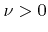. Thus the probability density function for the polar angle is given as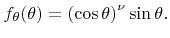(5.36)

Calculating the cumulative distribution function results in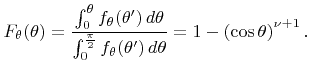(5.37)

A variate obeying an arbitrary distribution can be obtained using the inversion method . A uniformly distributed variate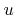on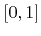mapped by the inverse cumulative distribution function leads to the desired distribution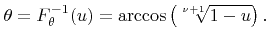(5.38)

Since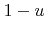is uniformly distributed onas well, the random polar angle can also be generated by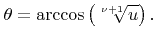(5.39)

Algorithm 5.2 summarizes the generation of a random polar angle following the probability density function (5.36).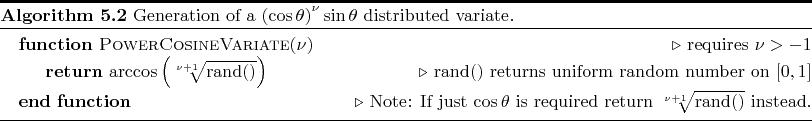Next: 5.3.2 Coned Cosine Distribution Up: 5.3 Generation of Random Previous: 5.3 Generation of Random

Otmar Ertl: Numerical Methods for Topography Simulation